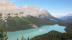## Bit string manipulation made easy with Bit::Manip

I've been writing a lot of software lately that deals with direct hardware access (specifically analog and digital hardware for the Raspberry Pi). This means that I've had to learn some C, as well as get proficient with bit manipulation and the bitwise operators.

As part of my learning, I thought I'd write a module to do this bit manipulation for me, hence Bit::Manip was born. (There's also a `Bit::Manip::PP` for those who can't/don't want to use XS. It should be indexed shortly).

Here's a scenario based example of how the software can be used.

You have a 16-bit configuration register for a piece of hardware that you want to configure and send in. Here's the bit configuration

``````|<--------- 16-bit config register ---------->|
|                             |               |
|---------------------------------------------|
|                             |               |
|                             |               |
|<------Byte 1: Control------>|<-Byte0: Data->|
|                             |               |
|-----------------------------|---------------|
| 15 | 14 13 | 12 11 | 10 9 8 | 7 6 5 4 3 2 1 |
__   _____   _____   ______   _____________
^      ^       ^        ^          ^
|      |       |        |          |
START    |       |      UNUSED      DATA
CHANNEL    |
PIN SELECT
``````

...and the bit configuration:

``````15:     Start conversation
00 - do nothing
01 - start conversation

14-13:  Channel selection
00 - channel 0
01 - channel 1
11 - both channels

12-11: Pin selection
00 - no pin
01 - pin 1
11 - pin 2

10-8:   Unused (Don't care bits)

7-0:    Data
``````

Let's start out with a 16-bit word, and set the start bit. Normally, we'd pass in an actual value as the first param (`\$data`), but we'll just set bit `15` on `0` to get our initial data.

``````my \$data = bit_on(0, 15);
``````

A couple of helper functions to verify that we indeed have a 16-bit integer, and that the correct bit was set:

``````say bit_count(\$data);
say bit_bin(\$data);
``````

Output to ensure we're good.

``````16
1000000000000000
``````

Now, we've got the conversation start bit set in our register, and we want to set the channel. Let's use both channels. For this, we need to set multiple bits at once. The datasheet says that the channel is at bits 14-13. Take the LSB (13), pass it along with the data to `bit_set()`, followed by the number of bits to update and as the last parameter, put the binary bit string that coincides with the option you want (`0b11` for both channels):

``````# setting channel

my \$bits_to_update = 2;
\$data = bit_set(\$data, 13, \$bits_to_update, 0b11);

# result: 1110000000000000
``````

We'll use pin 1, and per the datasheet, that's `0b01` starting from bit 11:

``````# setting pin

\$data = bit_set(\$data, 11, 2, 0b01);

# result: 1110100000000000
``````

The next two bits are unused, so we'll ignore them, and set the data. Let's use 186 as the data value (10111010 in binary):

``````# setting data

\$data = bit_set(\$data, 0, 8, 186);

# or: bit_set(\$data, 0, 8, 0b10111010);

# result: 1110100010111010
``````

Now we realize that we made a mistake above. We don't want both channels after all, we want to use only channel 1 (value: 0b01). Since we know exactly which bit we need to disable (14), we can just turn it off:

``````\$data = bit_off(\$data, 14);

# result: 1010100010111010
``````

(You could also use `bit_set()` to reset the entire channel register bits (14-13) like we did above).

Let's verify that we've got the register configured correctly before we send it to the hardware. We use `bit_get()` for this. The 2nd and 3rd parameters are MSB and LSB respectively, and in this case, we only want the value from that single bit:

``````my \$value = bit_get(\$data, 15, 15);
say bit_bin(\$value);

# result: 1
``````

So yep, our start bit is set. Let's verify the rest:

``````# data

# (note no LSB param. We're reading from bit 7 through to 0. LSB defaults
# to 0 if not sent in).

# since we readily know the data value in decimal (186), we don't need
# to worry about the binary representation

say bit_get(\$data, 7);

# result 186

# channel

say bit_bin(bit_get(\$data, 14, 13));

# result 1

# pin select

say bit_bin(bit_get(\$data, 12, 11));

# result 1

# ensure the unused bits weren't set

say bit_get(\$data, 10, 8);
``````

So now we've set up all of our register bits, and confirmed it's ready to be sent to the hardware for processing.Just Another Perl Hacker